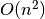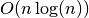# get_bonds¶

`Cartesian.``get_bonds`(modified_properties=None, maximum_edge_length=25, difference_edge=6, use_valency=False, use_lookup=False, set_lookup=True, divide_et_impera=True, atomic_radius_data='atomic_radius_cc')[source]

Returns a dictionary representing the bonds.

Warning

This function is not sideeffect free, since it assigns the output to a variable `self.__bond_dic` if `set_lookup` is `True` (which is the default). This is necessary for performance reasons.

The Cartesian().get_bonds() method will use or not use a lookup
depending on `use_lookup`. Greatly increases performance if True, but could introduce bugs in certain situations.

Just imagine a situation where the `Cartesian().frame` is changed manually. If you apply lateron a method e.g. `to_zmat()` that makes use of `get_bonds()` the dictionary of the bonds may not represent the actual situation anymore.

You have two possibilities to cope with this problem. Either you just re-execute `get_bonds` on your specific instance, or you change the `internally_use_lookup` option in the settings submodule. Please note that the internal use of the lookup variable greatly improves performance.

Parameters: modified_properties (dic) – If you want to change the van der Vaals radius or valency of one or more specific atoms, pass a dictionary that looks like: ```modified_properties = {index1 : {'atomic_radius' : 1.5, 'valency' : 8}, ...} ``` For global changes use the constants.py module. maximum_edge_length (float) – Maximum length of one edge of a if divide_et_impera is True. (cuboid) – difference_edge (float) – use_valency (bool) – If `True` atoms can’t have more bonds than their valency. This means that the bonds, exceeding the number of valency, with lowest overlap will be cut, although the van der Waals radii overlap. use_lookup (bool) – set_lookup (bool) – divide_et_impera (bool) – Since the calculation of overlaps or distances between atoms scale with, it is recommended to split the molecule in smaller cuboids and calculate the bonds in each cuboid. The scaling becomes then. This approach can lead to problems if `use_valency` is `True`. Bonds from one cuboid to another can not be counted for the valency.. This means that in certain situations some atoms can be oversaturated, although `use_valency` is `True`. atomic_radius_data (str) – Defines which column of `constants.elements` is used. The default is `atomic_radius_cc` and can be changed with `settings.atomic_radius_data`. Compare with `add_data()`. Dictionary mapping from an atom index to the indices of atoms bonded to. dict